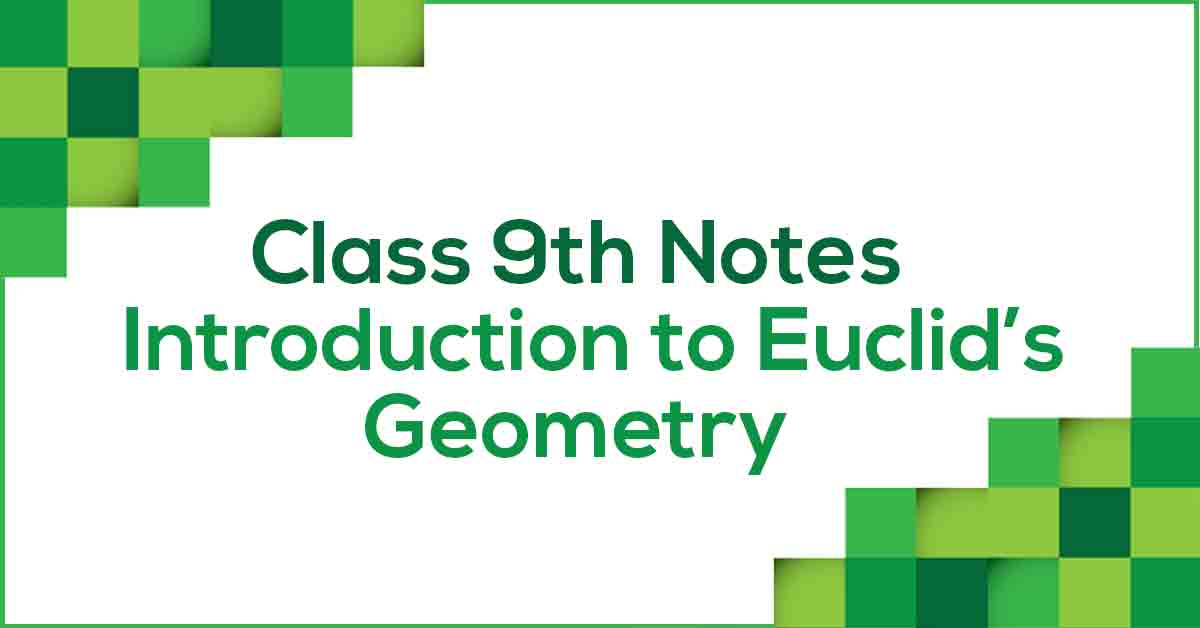Euclid’s Geometry Class 9 NotesClass 9 Maths Chapter 5 Introduction to Euclid’s Geometry Notes - PDF Download

Introduction to Euclid's Geometry Class 9 Notes are prepared by our panel of highly experienced teachers which not only reduces the pressure on the students but also offer them a simple way to study or revise the chapter. These notes are prepared by the teachers out of the past 10 years question papers so that the students don’t miss out on any of the important topics. Introduction to Euclid’s Geometry Class 9 Notes would fuel your exam preparation and increase your self-confidence.

Learn the introduction to Euclid's Geometry.

Euclid’ was a Greek mathematician regarded as the ‘Father of Modern Geometry’. Euclid introduced the fundamentals of geometry in his book called “Elements”. The postulates stated by Euclid are the foundation of Geometry and are rather simple observations in nature.

Learn more about these postulates and axioms in Introduction to Euclid’s Geometry Class 9 Notes pdf.